# What is a GEME Frequency Source?

To date, sources and detectors operating in 0.1 to 10 THz spectrum have been inefficient or too difficult to fabricate because the frequencies are too high for electronics or too slow for optical devices. This section of the spectrum is known as the Terahertz (THz) gap. Providing solutions for this gap can result in many interesting and useful applications for communications, scientific instrumentation, and high frequency imaging.

The Graphene Electromagnetic Emitter (GEME) has been designed to fill this THz gap. It is a new type of frequency source designed to produce terahertz frequencies based on a synchrotron architecture and a graphene Field Effect Transistor (FET). This frequency source produces a single output frequency ranging from 10 GHz to 1000 GHz (0.01 to 1 THz) with 1 mW of output power. The preliminary power dissipation is expected to be less than 20 W for a device of this size for any frequency in this range.

Figure 1 shows the a GEME frequency source with a 1 mm coaxial connector for an output frequency ranging to 110 GHz (left), and with a UG-387 style flange for WR-12 to WR-1 waveguides for an output frequency ranging from 60 GHz to 1100 GHz (right). Two feedthroughs provide a filtered power input to the module and an output power level control.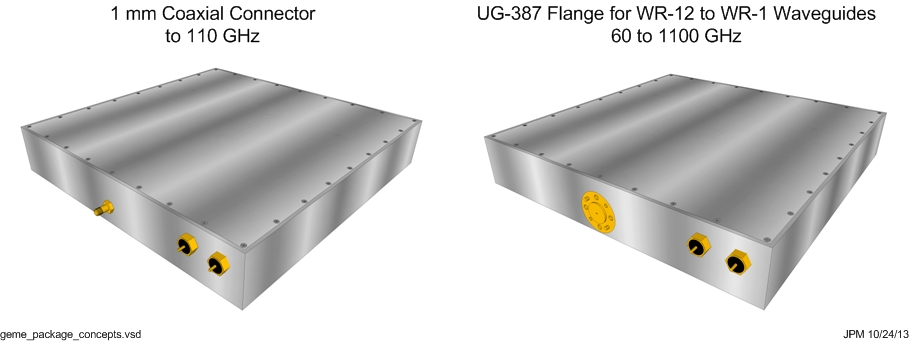Figure 1 GEME Frequency Source Package Concepts

Table 1 shows the preliminary performance specifications for a GEME frequency source.

Table 1 Preliminary GEME Performance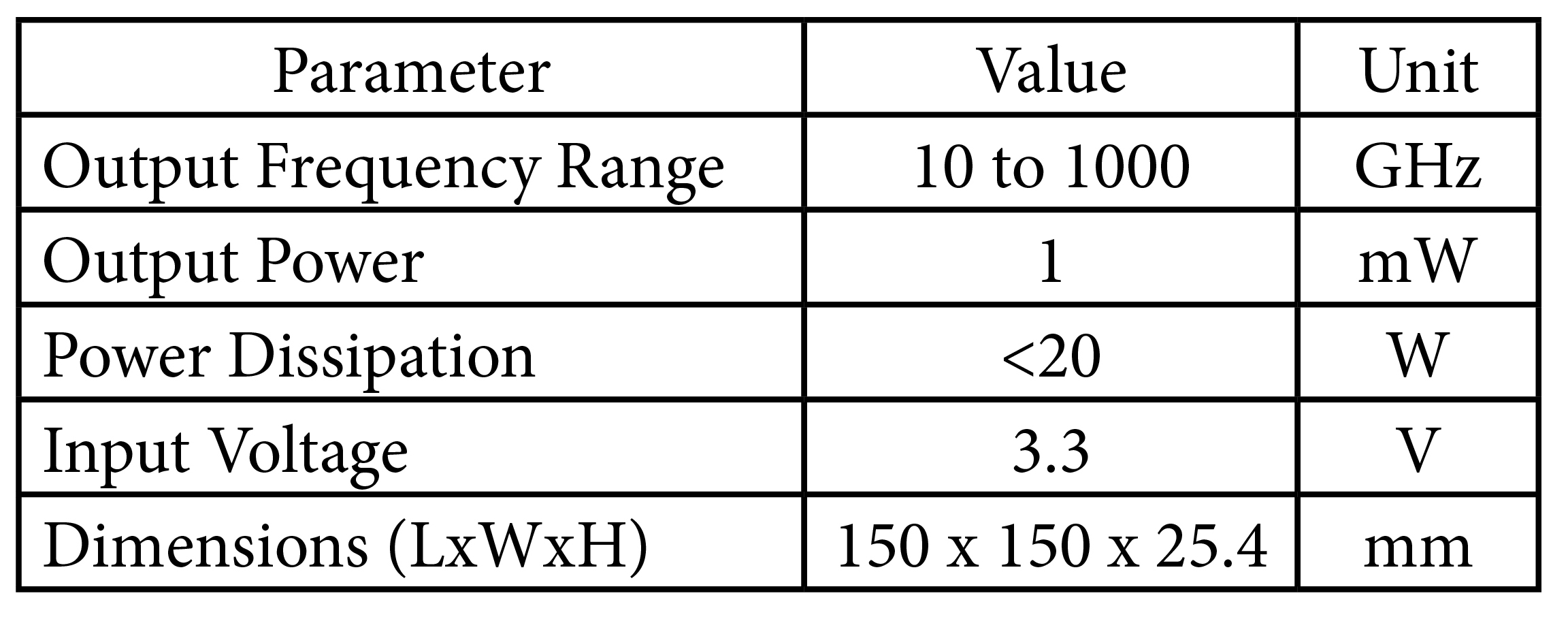# GEME Theory of Operation

## Graphene FET Assembly

Figure 2 shows an illustration of the Graphene Electromagnetic Emitter (GEME). The GEME is a graphene Field Effect Transistor (FET)  surrounded by a magnet array that produces a periodic alternating magnetic field that is perpendicular to the graphene channel. This alternating magnetic field produces an alternating force on the electrons traveling in the graphene channel of the FET causing the electronics to accelerate and emit electromagnetic wave. The frequency of the electromagnetic waves depends on the period of the magnet array and the velocity of the electrons in the graphene channel. The output power depends on the electron density and the area of the graphene channel(s).

The top part of Figure 2 shows the top view of the GEME in the x-y plane. The source, drain, and graphene channel can be seen along with the external power sources used to power the device. A voltage between the drain and source, Vds, provides the electromotive force to cause the electrons to flow between the source and the drain. The voltage between the gate and the source, Vgs, controls the number electrons in the channel.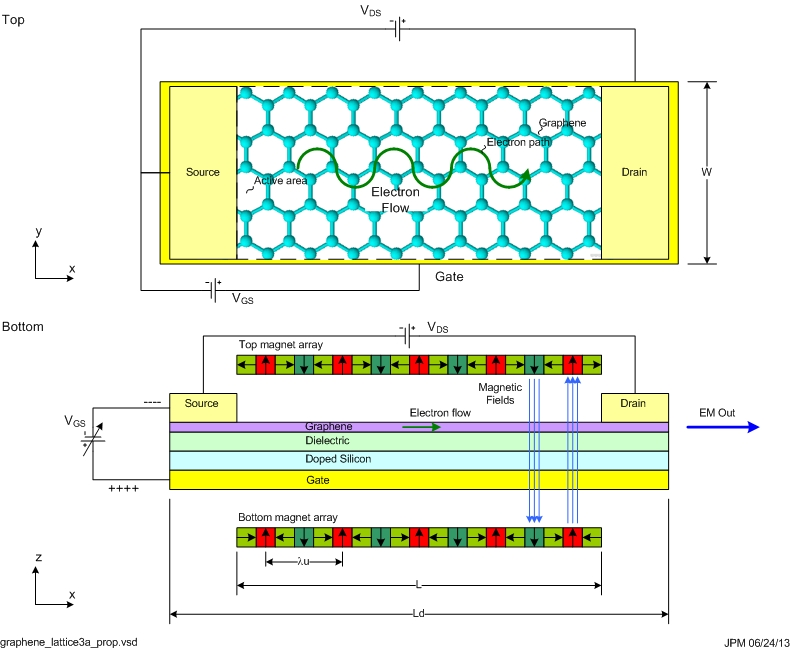Figure 2 Graphene EM Emitter Block Diagram

The bottom part of Figure 2 shows the side view of the GEME in the x-z plane. The FET structure is made up of a bottom metal, a doped silicon wafer, a dielectric layer, and a graphene layer. Contacts are deposited on the graphene to form the source and the drain. The bottom metal and dope silicon wafer form the gate of the device. The dielectric layer acts an insulator between the gate and the graphene layer. The graphene layer forms the field controlled conductive channel between the source and drain contacts. Increasing Vgs increases the electron density, increases the current flow, and increases the conductivity in the channel.

## Magnet Arrays

To simplify the processing of the prototype, the magnet arrays shown are external to the FET structure, and are made of individual magnets arranged to produce the desired periodic alternating magnetic field with the strongest magnetic field possible. The magnet arrays above and below the graphene channel produce a periodic alternating magnetic field that force the electrons to follow an undulating path from the source to the drain. The undulating motion of the electron produces the electromagnetic radiation [2, p. 135]. The magnets are arranged to produce an undulator using a Halback magnetic array . The top magnet array produces an alternating magnetic field facing the graphene layer. The bottom magnet array produces an alternating magnetic field facing the graphene layer. The period of the magnet arrays are [lambda]u (due to a website bug special characters are written out). This approach doubles the magnetic field between the magnet arrays producing a stronger force on the electrons in the graphene channel to increase the output power.

## Magnetic Fields

Figure 3 shows how the magnetic fields combine to produce no field on one side of the array and a double strength field on the other. The arrow shows the magnetization direction with the arrow head pointing North. In the top magnet array, the magnets point left, up, right, down, left, up, right, and so on across the array. The magnetic fields of the up and down magnets couple together and are space to alternate between left-right magnets. The left-right magnets between them cancel the magnetic fields on top of the array and re-enforce the magnetic fields below the array. Thus, the fields below the array double in strength while the fields on top of the array cancel out.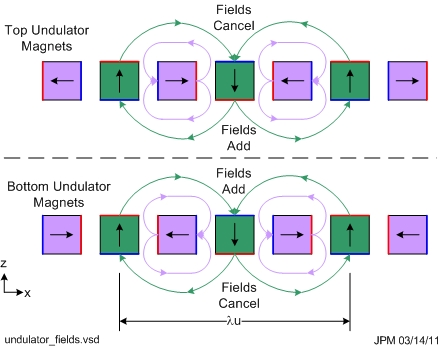Figure 3 Halback Magnet Array Field Diagram

The bottom array works in a similar way except that the magnets are arranged so that the magnetic fields add on the top of the array and cancel at the bottom of the array. This configuration is known as an Halback array named after Klause Halback .

Figure 4 shows alignment of the top and bottom magnet arrays. The magnetizations of the up and down fields in the z-direction align with each other so that the electrons in the graphene channel experience magnetic fields of alternating direction. The magnetic fields produce a periodic Lorentz force that causes the electrons to follow a sinusoidal-like path in the y-direction. This sinusoidal force subjects the electrons to an acceleration which causes them to emit EM radiation.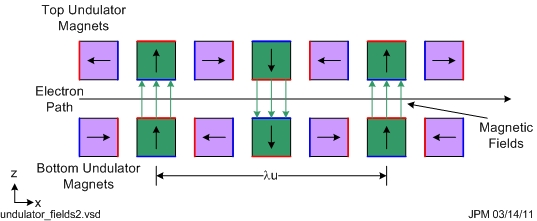Figure 4 Halback Magnet Array Perpendicular Fields Diagram

Figure 5 show a 3-D illustration of the two magnet assemblies and the sinusoidal electron path in the graphene channel.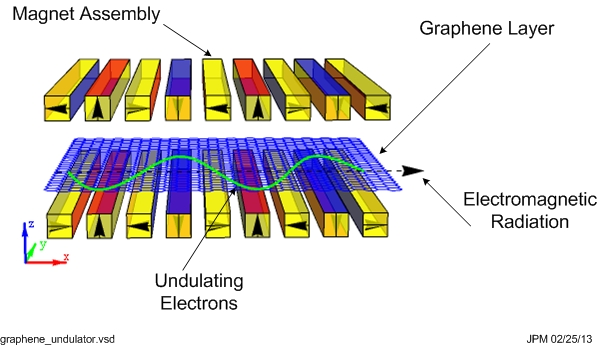Figure 5 Magnetic Array with Graphene Layer and Electron Path Illustration

## Introduction to the Calculations

A force is needed to accelerate electrons so that they produce electromagnetic radiation. The GEME FET assembly and magnet arrays are designed with a specific geometry to apply and maximize this force (known as the Lorentz force). This force has two components. The first component comes from the electric field produced by the drain-source voltage (Vds). Vds produces an electric field (Ex) across the graphene channel and causes the electrons to flow between the source and the drain in the x-direction. The electron velocity (Vx) depends on the electric field (Ex). The magnitude of this component of the force is dependent on the electron charge (Q), the length of the graphene channel (L), and drain-source voltage Vds. The second component comes from the periodic magnetic field (Bz) produced by the magnet arrays, the electron velocity (Vx), and the electron charge (Q). Moving electrons in a magnetic field experience a force that is perpendicular to the velocity and magnetic field. The geometry of the device makes it so the force is applied only in the plane of the graphene channel in the ±y-direction so the electrons are pushed back and forth as they flow past the alternating north and south poles of the magnet arrays. Once the force is known, the acceleration (a) can be found from F=a Me where F is the Lorentz force and Me is the effective mass of the electron. Graphene has the interesting property that the effective mass of the electron depends on the number of electrons present in the graphene channel. So the acceleration gets stronger with fewer electrons (lower electron density).

The radiated field pattern looks like a donut (toroid). The instantaneous power emitted from this pattern is calculated using the Poynting vector. The Poynting vector shows the magnitude and direction of the energy flowing from the electron in Watts/cm2. Integrating this power over the area of a sphere surrounding the electron provides the total instantaneous power radiated (P). The average power is the found by computing the instantaneous power of a whole cycle.

There are several electron velocities in graphene. The Fermi velocity is determined by the lattice geometry of the A and B atoms. This is the maximum theoretical electron velocity. The electrons in graphene interact with the material it is in contact with (SiO2 in this case) and slow down or saturate due to electron-phonon interactions in the SiO2. This is known as the saturation velocity (Vsat) The electron velocity slows down further and develops a drift velocity. This drift velocity (Vx) is used to calculate the acceleration of the electron. The mobility and the electric field (Ex) affect the electron velocity (Vx) so the higher the mobility the higher the overall electron velocity. A higher electric field will produce a higher the electron drift velocity until the saturation velocity is reached.

The gate and graphene form plates of a capacitor separated by the gate dielectric (SiO2). This gate capacitance is determined by the thickness (t) of the gate dielectric and the average relative dielectric constant (kappa) of the gate dielectric. The graphene layer also acts as capacitor by itself. This capacitance is known as the quantum capacitance and adds in series with the gate capacitance. The quantum capacitance also produces its own quantum carrier density that adds to the overall carrier density produced by the application of a gate voltage. These quantities depend on the gate capacitance (Cg), gate voltage (Vgs), the charge of an electron (Q), Plank’s reduced constant (ħ), and the Fermi Velocity (Vf).

The total output power (Pn) is found by multiplying the power radiated by an electron (P) times the number of electrons (n) for electrons that radiate with electric and magnetic fields with no special relationship to each other. If the electrons radiate with fields that are correlated, then the total output power (Pn) is found by multiplying the power radiated by an electron (P) times the number of electrons squared (n2). The frequency emitted is determined by the electron velocity (Vx) divided by the length of the periodic magnetic field [lambda]u. The calculation of these parameters follows below in more detail.

## Lorentz Force

With this geometry, the Lorentz forces on the electrons cause them to deviate back and fourth in the y-direction producing a sinusoidal-like path. The Lorentz force is shown in the equation below in equation (1) [5, pp. 13–1].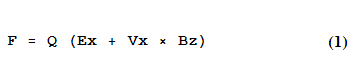Q is the charge of an electron (Q=qe=-1.6 * 10-19 C), Vx is the velocity of the electron in the x-direction, and Bz is the magnetic field in the z-direction. Ex is the electric field in the minus x-direction and is calculated from the geometry of the device as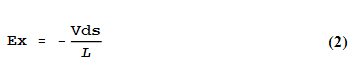Where Vds is the drain-source voltage and L is the length of the graphene channel. The electric field provides the force the drives the electrons to flow and influences the velocity of the electrons in the graphene layer. Since Vx and Bz are perpendicular to each other equation (1) simplifies tosince the cross product contains the term Sin(90°)=1. The magnetic field, Bz, is perpendicular to the velocity direction by the geometry of the device and is periodic with x by the arrangement of magnets in the Halback magnet arrays as follows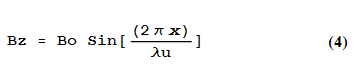Bo is the nominal strength of the magnet at the up or down location of the magnet array.

## Electron Acceleration

The sinusoidal path subjects the electrons to an acceleration that can be found by solving F=a Me and substituting the force found in equation (3). The result is shown in equation (5) where Me is the effective mass of an electron in graphene which is carrier density dependent in graphene [7, p. 113].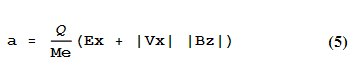An accelerating charge will then emit EM radiation. The acceleration causes distortions in the electric field around the charge. These field distortions are the source of the EM radiation [8, p. 270]. This acceleration is also perpendicular to the velocity so the radiation pattern has a toroid or donut shape oriented so that the donut hole aligns with the direction of acceleration, as shown in Figure 6 [2, p. 37]. As the electron flows along the sinusoidal path in the graphene layer, the radiation pattern looks like a donut or wheel rolling along the sinusoidal path with the donut hole or axle being parallel to the graphene layer.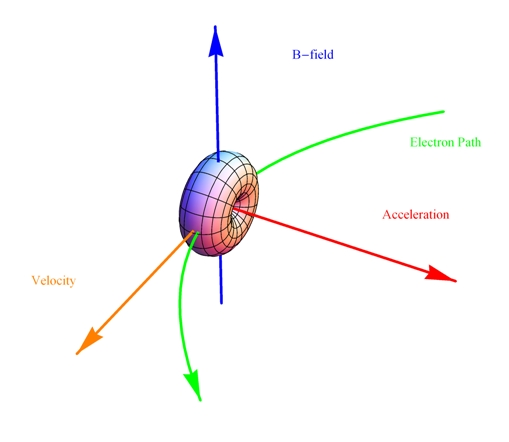Figure 6 Electron Radiation Pattern Illustration

The total instantaneous power radiated from the electron is computed using the Poynting vector (S=E×H) and integrated around the solid angle of the radiation [2, p. 37].Where [epsilon]0 is the Permittivity of the vacuum and c is the speed of light. Total average power is found by averaging over one cycle and found as P/2. The radiated power from a single electron is very small because the acceleration is small due to the non-relativistic electron velocity, but the structure has three key design parameters that allow the production of higher output power.

The first is high electron densities (that are controlled by applying higher gate-source voltages to a limit), the second is by increasing the active area (graphene channel area) of the device (W * L), and third is by increasing the number of graphene layers. The limitation on electron density occurs because at some point the higher carrier density begins to impede the electron velocity, which begins to limit the acceleration and dim the output power. In this case, too many electrons are getting in the way of each other, so the electron velocity begins to drop due to increased electron-electron scattering and other scattering processes. Electron densities of greater than 1012 /cm2 have been described in the literature [9, p. 7].

## Electron Velocity

The Fermi electron velocity through graphene is determined by the honeycomb geometry of graphene and the [pi]-orbitals of the carbon, and is reported as 1.00337 * 108 cm/second [9, p. 4][6, p. 1]. The Fermi velocity is the maximum electron velocity in graphene. Electrons are scattered by phonons that are present in the dielectric that the graphene is deposited on, which gives rise to a saturation velocity [6, p. 2]. The saturation velocity is dependent on the electron density and phonon energy level in the dielectric, which ranges from 55 meV to 238 meV for SiO2 and other dielectrics) [10, p. 2]. The saturation velocity is used to determine the drift velocity, Vx, as shown by equation (7) [11, p. 4].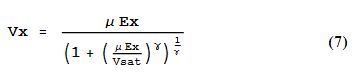Where [mu] is the mobility of the graphene layer, Ex is the electric field strength, and [gamma] is a fitting parameter that is approximately 2 for electrons in graphene [11, p. 5]. The peak electron drift velocity, Vx, occurs when the electron density just reaches saturation for a given electric field strength.

## Gate Capacitance

To compute the electron density, the gate capacitance needs to be calculated. The gate capacitance is determined by the properties of the gate dielectric materials itself (such as SiO2). The gate dielectric material capacitance is the ratio of the dielectric constant divided by the gate thickness [12, p. 3].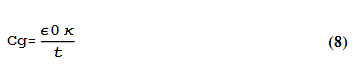Where [epsilon]0 is the Permittivity of the vacuum, [kappa] is the relative dielectric constant of the dielectric (3.9 for SiO2), and t is the gate dielectric thickness. The typical dielectric thickness of a silicon wafer with a SiO2) layer is 300 nm. The average gate capacitance for a 300 nm SiO2 layer is 7.23 * 10-9 Farads/cm2, for example [9, p. 13].

## Electron Density

With the gate capacitance available, the carrier density, n, can be computed [9, p. 13].Where Vgs is the gate-to-source voltage and nq is the quantum carrier density. The quantum carrier density is a function of the gate capacitance and carrier Fermi velocity, Vf, but it is in general so small for large devices that the carrier density is approximated as n=(Cg Vg)/Q [9, p. 13].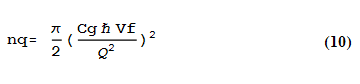Where Vf is the Fermi velocity, and [h bar] is Planck’s constant divided by 2[pi]. The carrier density has been reported to range from 109 /cm2 to 5*1012 /cm2 in the literature [9, p. 7].

## Total Average Power

With the carrier density, the total average output power is power output from one electron times the total number of electrons in the graphene as calculated in equation (11).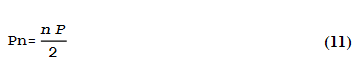If the motions of the accelerated electrons have no special relationship or are uncorrelated, then the output power scales with the number of electrons as shown in equation (11). This power is estimated at 1 mW for a device built on a 100 mm wafer. If, however, the electrons are phase correlated so that the E and B fields are correlated, then the output power scales as the number or electrons squared (n2 instead of n as shown in equation (12)). The output power is estimated to be in the 100 W range in this case with a much smaller carrier density. The interactions of the electrons with each other, with impurities, and with the geometry of the device are very complex so it is unclear how the electrons will behave on average. One of the objectives of the proposal will focus on this behavior.The carrier density is a function of gate voltage, so the output power can be controlled by varying the gate voltage. A positive gate voltage produces electrons in the graphene layer, and a negative gate voltage produce holes (positive charge) in the graphene layer.

## Output Frequency

The emitted frequency is related to the velocity of the electron divided by the period of the undulator magnet assembly, as shown in equation (12) [13, p. 59]. This frequency, fu, is the fundamental frequency that is radiated.The analysis presented above describes the continuous and uniform acceleration of a large number of electrons in the graphene that emit EM radiation. This EM emission can be interfered with by impurities, and defects on the graphene or in dielectric layers around the graphene. Thus careful processing and materials selection is needed to optimize the electron velocity. The EM emissions from the device occurs from the use of DC voltages and do not require complex oscillators to produce this EM radiation. Making the magnet array periods smaller can produce higher frequencies. In theory, frequency sources with output frequencies of Terahertz or more should be possible.

See my blog Graphene FET Toy Physics Model to interactively explore graphene FET physics such as electron velocity, gate capacitance, and electron density.

 F. Schwierz, “Graphene transistors,” Nat. Nanotechnol., vol. 5, no. 7, pp. 487–496, 2010.
 D. Attwood, Soft X-Rays and Extreme Ultraviolet Radiation: Principles and Applications, 1st ed. Cambridge University Press, 2007.
 “Undulator,” Wikipedia, the free encyclopedia. 27-Feb-2013.
 “Halbach array,” Wikipedia, the free encyclopedia. 23-Apr-2013.
 R. P. Feynman, The Feynman Lectures on Physics (3 Volume Set). Addison Wesley Longman, 1970.
 V. Perebeinos and P. Avouris, “Inelastic scattering and current saturation in graphene,” Phys. Rev. B, vol. 81, no. 19, p. 195442, May 2010.
 A. H. C. Neto, F. Guinea, N. M. R. Peres, K. S. Novoselov, and A. K. Geim, “The electronic properties of graphene,” arXiv: 0709.1163, Sep. 2007.
 M. A. (Mar. A. H. Heald and J. B. Marion, Classical Electromagnetic Radiation, 003 ed. Brooks Cole, 1994.
 S. D. Sarma, S. Adam, E. H. Hwang, and E. Rossi, “Electronic transport in two dimensional graphene,” arXiv, vol. 1003.
 V. Perebeinos and P. Avouris, “Current Saturation and Surface Polar Phonon Scattering in Graphene,” 0910.4665, Oct. 2009.
 V. E. Dorgan, M.-H. Bae, and E. Pop, “Mobility and Saturation Velocity in Graphene on SiO2,” 1005.2711, May 2010.
 A. Barreiro, M. Lazzeri, J. Moser, F. Mauri, and A. Bachtold, “Transport Properties of Graphene in the High-Current Limit,” Phys. Rev. Lett., vol. 103, no. 7, p. 076601, 2009.
 A. Hofmann, The Physics of Synchrotron Radiation, 1st ed. Cambridge University Press, 2007.

# Variable Glossary

Table 3 shows a description of the variables used above.

Table 3 Variable Glossary Table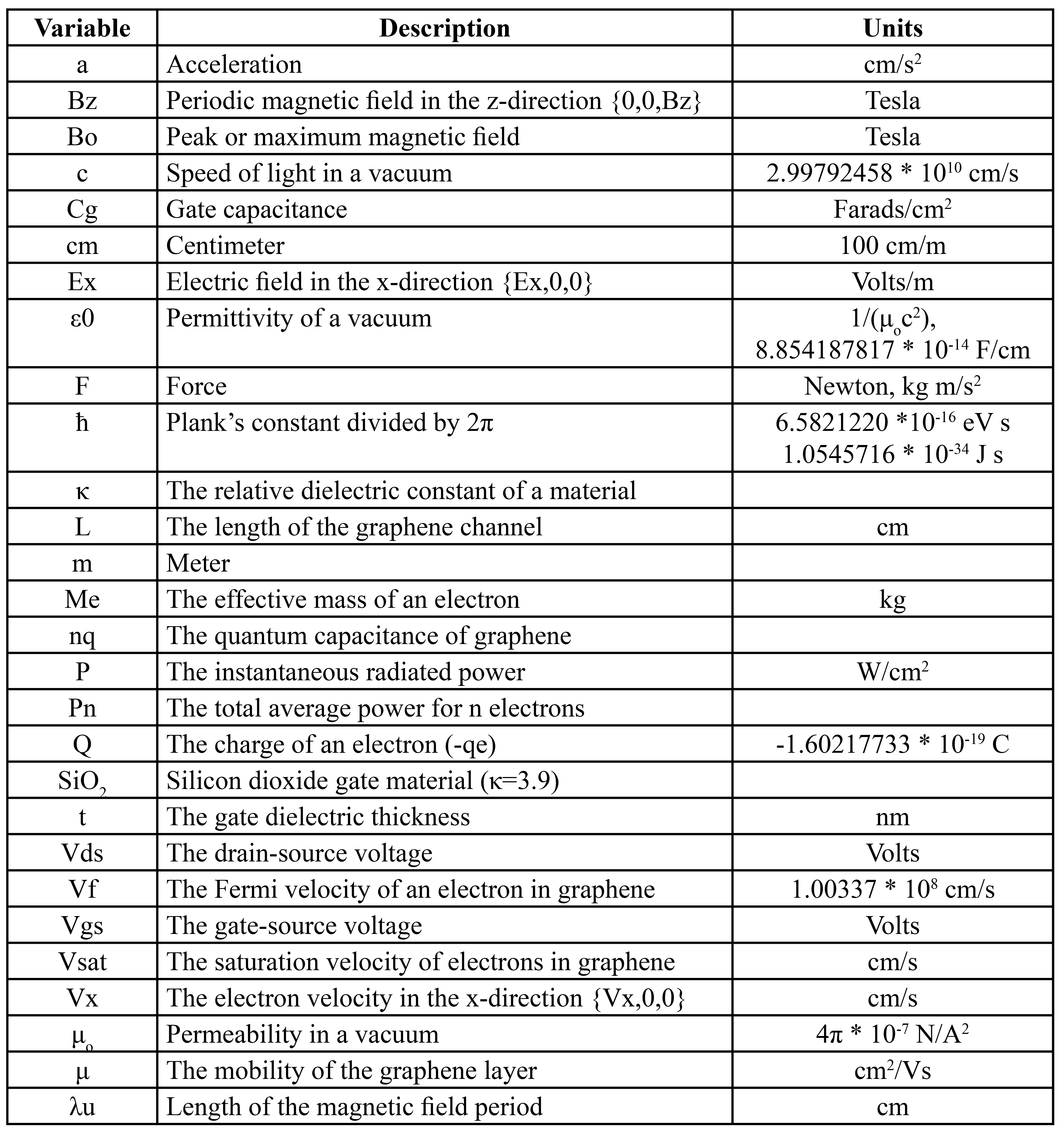# Units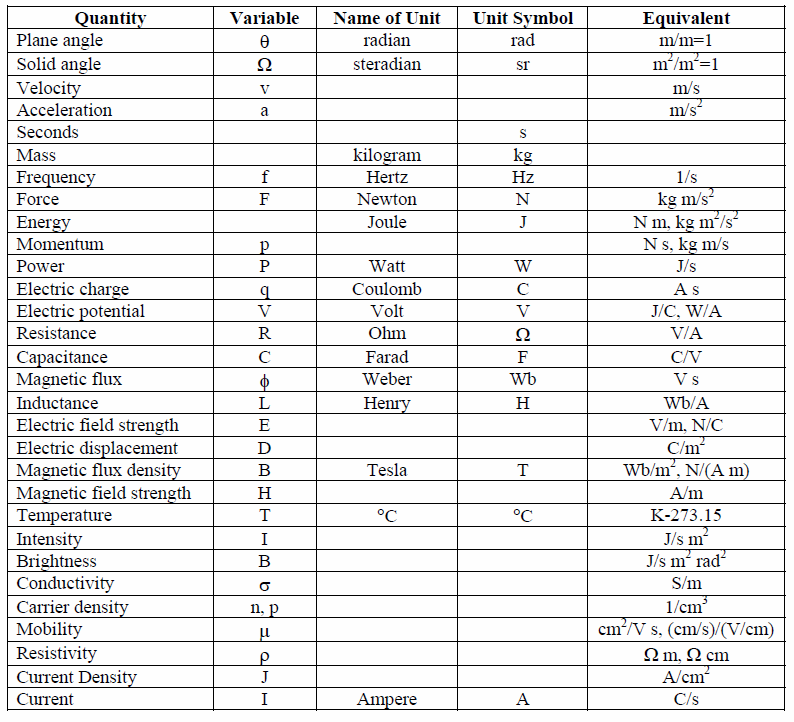# Constants and Conversionsp-brane LLC Proprietary

www.p-brane.com